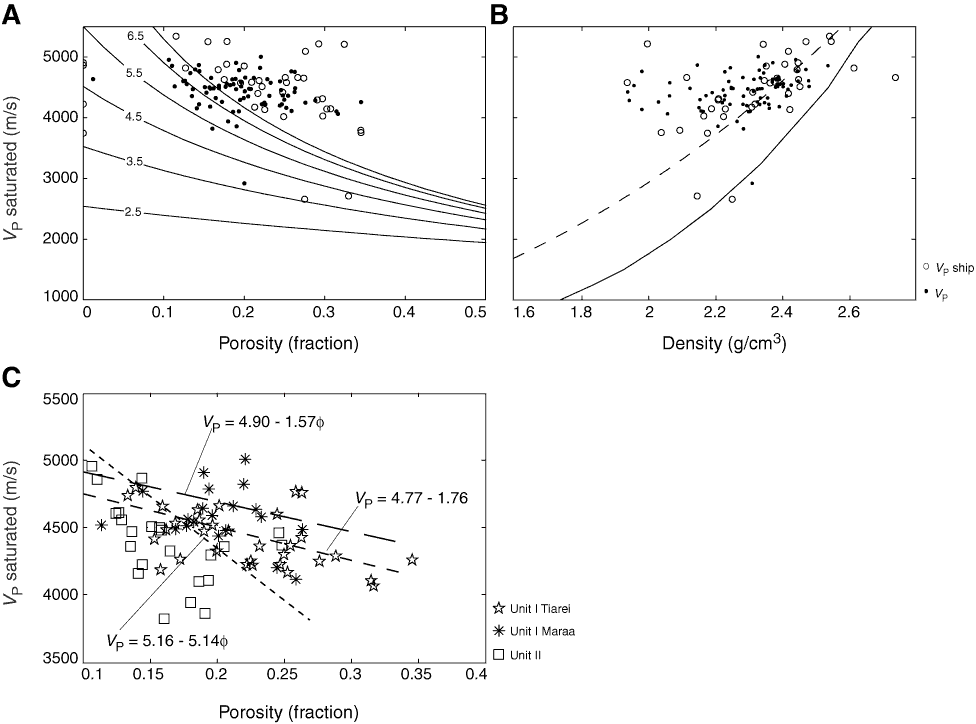Figure F9. Cross-plots of acoustic velocity vs. porosity and density undifferentiated for mineralogy. A. Velocity vs. porosity with time-average transforms of Wyllie et al. (1956) for various matrix velocities (solid lines). B. Velocity vs. density with Gardner’s equation (1974) for limestones (solid line) and Anselmetti-Eberli equation (1993) (dashed line). C. Velocity vs. porosity with best-fit linear regression lines plotted for all three sample groups. Large dashed line = Unit I, Maraa; medium dashed line = Unit I, Tiarei; small dashed line = Unit II.